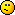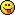Discuss Scratch

The shortest FizzBuzz YOU can make!

This is a challenge for yourself to make the shortest FizzBuzz you can!
Here's my one in Python 3:
[(str(i) if (i%3 and i%5) else "")+("Fizz" if not i%3 else "")+("Buzz" if not i%5 else "") for i in range(1, 100)]
I'm interested in the different language people will choose.

Game Over
You'll find me on @LastContinue from now on.

The shortest FizzBuzz YOU can make!

bybb wrote:

–snip–
Wouldn't you have to put
print
in the beginning of your code for it to output the test though?
Or is outputting not really necessary, just the calculation?The shortest FizzBuzz YOU can make!

Wettining wrote:

bybb wrote:

–snip–
Wouldn't you have to put
print
in the beginning of your code for it to output the test though?
Or is outputting not really necessary, just the calculation?
Depends on how you run it.
If you run it as a py files, yes you'd need print.
If you run it in IDLE (or in python) it prints the output by default.

Game Over
You'll find me on @LastContinue from now on.

The shortest FizzBuzz YOU can make!

BRB, gonna write my solution in PiedPiper… https://scratch.mit.edu/projects/117951730/

It's been a while, I'm not sure if I have any documentation for it even…

In the meantime, here's my python3 solution:

["Fizz"*(1-x%3)+"Buzz"*(1-x%5)or str(x)for x in range(1,100)]

Last edited by novice27b (June 9, 2018 15:48:24)

i use arch btw

The shortest FizzBuzz YOU can make!

setMy 'Output' ''
for i 100 {
if ((i % 3) == 0) {
setMy 'Output' (join 'Fizz' (join ',' (newline)) (toString (my 'Output')))
} ((i % 5) == 0) {
setMy 'Output' (join 'Buzz' (join ',' (newline)) (toString (my 'Output')))
} else {
setMy 'Output' (join (toString i) (join ',' (newline)) (toString (my 'Output')))
}
}

(To run this you have to run the project in GP, download the project here)The shortest FizzBuzz YOU can make!

JavaScript:
new Array(100).fill('').map((v,i)=>(++i%3?v:'Fizz')+(i%5?v:'Buzz')||i)

- Sheep_maker This is a kumquat-free signature. :P
This, my signature, appears below all my posts. Discuss it on my profile, not the forums. Here's how to make your own.
Post count: more than 6
.postsignature { overflow: auto; } .scratchblocks { overflow-x: auto; overflow-y: hidden; }

This will return false in your favourite programming language (…except Scratch):
(1.0 / 5e-324 - 1.0 / 5e-324) == (1.0 / 5e-324 - 1.0 / 5e-324)

The shortest FizzBuzz YOU can make!

C:
#include <stdio.h>
int main(boop)
{
int i;
for(i = 1; i <= 100; ++i)
{
if (i % 3 == 0)
printf("Fizz");
if (i % 5 == 0)
printf("Buzz");
if ((i % 3 != 0) && (i % 5 != 0))
printf("%d", i);
printf("\n");
}
return 0;
}
C++:
#include <iostream>
int main() {
for (int i = 1; i <= 100; i++) {
if (i%5==0) std::cout << "Buzz" << std::endl;
else if (i%3==0) std::cout << "Fizz" << std::endl;
else std::cout << i << std::endl;
}
return 0;
}

Last edited by Wettining (June 9, 2018 20:24:17)The shortest FizzBuzz YOU can make!

Wettining wrote:

C:
#include <stdio.h>
int main(boop)
{
int i;
for(i = 1; i <= 100; ++i)
{
if (i % 3 == 0)
printf("Fizz");
if (i % 5 == 0)
printf("Buzz");
if ((i % 3 != 0) && (i % 5 != 0))
printf("%d", i);
printf("\n");
}
return 0;
}
printf is slow cout is better.
#include <iostream>
using namespace std;
int main() {
for (int i=1;i<100;i++) {
if (i%3==0)
cout << "Fizz";
if (i%5==0)
cout << "Buzz";
if (i%3&&i%5)
cout << i;
cout << "\n";
}
return 0;
}

Game Over
You'll find me on @LastContinue from now on.

The shortest FizzBuzz YOU can make!

Wettining wrote:

C:
#include <stdio.h>
int main(boop)
{
int i;
for(i = 1; i <= 100; ++i)
{
if (i % 3 == 0)
printf("Fizz");
if (i % 5 == 0)
printf("Buzz");
if ((i % 3 != 0) && (i % 5 != 0))
printf("%d", i);
printf("\n");
}
return 0;
}
C++:
#include <iostream>
int main() {
for (int i = 1; i <= 100; i++) {
if (i%5==0) std::cout << "Buzz" << std::endl;
else if (i%3==0) std::cout << "Fizz" << std::endl;
else std::cout << i << std::endl;
}
return 0;
}
That fizzbuzz isn't correct since it will never output “FizzBuzz” on one line and also std::endl causes a delay just use \n in the stringGame Over
You'll find me on @LastContinue from now on.

The shortest FizzBuzz YOU can make!

bybb wrote:

also std::endl causes a delay
“a delay”

\$(".box-head").textContent = "committing AT crimes since \$whenever"

The shortest FizzBuzz YOU can make!

MegaApuTurkUltra wrote:

bybb wrote:

also std::endl causes a delay
“a delay”
https://stackoverflow.com/a/35581029
stream << std::endl is actually stream << ‘\n’ << std::flush. Explicit flushing has performance drawbacks, and this is why you shouldn't use it in performance-critical situations. You rarely think about such negligible performance issues while debugging, so it actualy is a good practice to explicitly flush debug output.

Game Over
You'll find me on @LastContinue from now on.

The shortest FizzBuzz YOU can make!

bybb wrote:

MegaApuTurkUltra wrote:

bybb wrote:

also std::endl causes a delay
“a delay”
snip
Sorry, I assumed you didn't actually know what endl was actually doing when you said it was just “a delay” so I was commenting on that. Looks like you do know what you're talking about though\$(".box-head").textContent = "committing AT crimes since \$whenever"

The shortest FizzBuzz YOU can make!

MegaApuTurkUltra wrote:

bybb wrote:

MegaApuTurkUltra wrote:

bybb wrote:

also std::endl causes a delay
“a delay”
snip
Sorry, I assumed you didn't actually know what endl was actually doing when you said it was just “a delay” so I was commenting on that. Looks like you do know what you're talking about thoughI first discovered it had some sort of delay when i was making a C++ program I could call from within a batch file to print coloured text within Windows, any text printed without the newline argument passed to my program would complete and print the next bit of text instantly, but when the newline flag was passed it had a delay, I did some research and learnt about how it was the equivalent of a newline and a buffer flush, rather than just a newline.

This is one of the best examples of my why I love programming so much, you can be doing something just for fun and end up learning half a dozen new things you wouldn't have otherwise.

Game Over
You'll find me on @LastContinue from now on.

The shortest FizzBuzz YOU can make!

bybb wrote:

[(str(i) if (i%3 and i%5) else "")+("Fizz" if not i%3 else "")+("Buzz" if not i%5 else "") for i in range(1, 100)]
you can remove a fair bit of whitespace from that…

anyway here's mine in Dolphin Smalltalk 7 (179 bytes)
Transcript show.1to:100do:[:i|Transcript show:(i\\3=0ifTrue:['Fizz'])displayString;show:(i\\5=0ifTrue:['Buzz'])displayString;show:(((i\\3>0)and:[i\\5>0])ifTrue:[i])displayString;cr]

ungolfed:
Transcript show.
1 to: 100 do:
[:i |
Transcript
show: (i \\ 3 = 0 ifTrue: ['Fizz']) displayString;
show: (i \\ 5 = 0 ifTrue: ['Buzz']) displayString;
show: (((i \\ 3 > 0) and: [i \\ 5 > 0]) ifTrue: [i]) displayString;
cr
]

if we remove the need to show the transcript and print newlines we can reduce it to 161 bytes!

1to:100do:[:i|Transcript show:(i\\3=0ifTrue:['Fizz'])displayString;show:(i\\5=0ifTrue:['Buzz'])displayString;show:(((i\\3>0)and:[i\\5>0])ifTrue:[i])displayString]

edit: this is shorter

Transcript show.1to:100do:[:i|Transcript show:(i\\15=0ifTrue:['FizzBuzz']ifFalse:[i\\5=0ifTrue:['Buzz']ifFalse:[i\\3=0ifTrue:['Fizz']ifFalse:[i displayString]]]);cr]

Last edited by xn--cr8h (June 10, 2018 03:30:03)

rip scratch forums 2007-2017

The shortest FizzBuzz YOU can make!

bybb wrote:

Wettining wrote:

C:
#include <stdio.h>
int main(boop)
{
int i;
for(i = 1; i <= 100; ++i)
{
if (i % 3 == 0)
printf("Fizz");
if (i % 5 == 0)
printf("Buzz");
if ((i % 3 != 0) && (i % 5 != 0))
printf("%d", i);
printf("\n");
}
return 0;
}
printf is slow cout is better.
#include <iostream>
using namespace std;
int main() {
for (int i=1;i<100;i++) {
if (i%3==0)
cout << "Fizz";
if (i%5==0)
cout << "Buzz";
if (i%3&&i%5)
cout << i;
cout << "\n";
}
return 0;
}
It's C not C++ lolThe shortest FizzBuzz YOU can make!

I wanted to do it in Batch but I didn't realise Batch actually had a modulo operator… Oh well.
@echo off

goto start

:modulo
set /a return=%1
set /a divide=%2
:loop_2
if %return% gtr 0 (
set /a return=%return%-%divide%
goto loop_2
)
exit /b

:start
set /a i=1
set /a return=0
:loop_1
set "fb="
set setted=0
call :modulo %i% 3
if %return% equ 0 (
set fb=%fb%Fizz
set setted=1
)
call :modulo %i% 5
if %return% equ 0 (
set fb=%fb%Buzz
set setted=1
)
if %setted% neq 1 set fb=%i%
echo %fb%
set /a i=%i%+1
if %i% equ 101 goto eol_1
goto loop_1
:eol_1
exit /b

Game Over
You'll find me on @LastContinue from now on.

The shortest FizzBuzz YOU can make!

bybb wrote:

I wanted to do it in Batch but I didn't realise Batch actually had a modulo operator… Oh well.
Just Windows Things™

#!/bin/bash
for i in {1..100}; do
case "\$((i % 3))\$((i % 5))" in
00) echo FizzBuzz;;
0*) echo Fizz;;
*0) echo Buzz;;
*) echo \$i
esac
done

\$(".box-head").textContent = "committing AT crimes since \$whenever"

The shortest FizzBuzz YOU can make!

bybb wrote:

Wettining wrote:

C:
#include <stdio.h>
int main(boop)
{
int i;
for(i = 1; i <= 100; ++i)
{
if (i % 3 == 0)
printf("Fizz");
if (i % 5 == 0)
printf("Buzz");
if ((i % 3 != 0) && (i % 5 != 0))
printf("%d", i);
printf("\n");
}
return 0;
}
printf is slow cout is better.
#include <iostream>
using namespace std;
int main() {
for (int i=1;i<100;i++) {
if (i%3==0)
cout << "Fizz";
if (i%5==0)
cout << "Buzz";
if (i%3&&i%5)
cout << i;
cout << "\n";
}
return 0;
}
You can make this shorter by replacing `using namespace std` with `using std::cout`.

Long live Kyoto Animation!

The shortest FizzBuzz YOU can make!

TheAspiringHacker wrote:

bybb wrote:

Wettining wrote:

C:
#include <stdio.h>
int main(boop)
{
int i;
for(i = 1; i <= 100; ++i)
{
if (i % 3 == 0)
printf("Fizz");
if (i % 5 == 0)
printf("Buzz");
if ((i % 3 != 0) && (i % 5 != 0))
printf("%d", i);
printf("\n");
}
return 0;
}
printf is slow cout is better.
#include <iostream>
using namespace std;
int main() {
for (int i=1;i<100;i++) {
if (i%3==0)
cout << "Fizz";
if (i%5==0)
cout << "Buzz";
if (i%3&&i%5)
cout << i;
cout << "\n";
}
return 0;
}
You can make this shorter by replacing `using namespace std` with `using std::cout`.
you can also make this shorter by not using 8 HECKING SPACES to indent

rip scratch forums 2007-2017

The shortest FizzBuzz YOU can make!

xn--cr8h wrote:

TheAspiringHacker wrote:

snip
you can also make this shorter by not using 8 HECKING SPACES to indent
Those are tab characters, not sets of 8 spaces.

- Sheep_maker This is a kumquat-free signature. :P
This, my signature, appears below all my posts. Discuss it on my profile, not the forums. Here's how to make your own.
Post count: more than 6
.postsignature { overflow: auto; } .scratchblocks { overflow-x: auto; overflow-y: hidden; }

This will return false in your favourite programming language (…except Scratch):
(1.0 / 5e-324 - 1.0 / 5e-324) == (1.0 / 5e-324 - 1.0 / 5e-324)